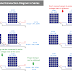In this article, we are going to learn how to connect Solar Panels in Series. We will learn what happens if we connect multiple solar panels in series if they have the same ratings. Also, we will learn what happens if we connect multiple solar panels in series if they have different ratings(means voltage, current, and power).

In Solar power generating systems or Solar Installation for domestic purposes such as in our home, sometimes we need to connect multiple solar panels in series. For example, Solar panels have a certain voltage at which they operate optimally. By connecting panels in series, you can increase the overall system voltage to match the requirements of a higher-voltage inverter or battery bank.

There are also some advantages of connecting solar panels in series such as, When panels are connected in series, the current remains the same as that of an individual panel. Lower currents can reduce energy losses due to resistance in wiring and connections. Even if the available space or budget is limited, you might need to use fewer panels with higher voltage outputs. Connecting panels in series can help achieve the desired system voltage with fewer panels.

## Solar Panel Connection in Series with Same Ratings

When we connect multiple solar panels having the same ratings in series,
1. The total output voltage will be the total or summation of the output voltage of individual solar panels.
2. The total output current will be the same as the current rating of the individual solar panel.
3. The total output power will be the total or summation of the output power of individual solar panels. That is the multiplication of Output Voltage and Output Current.

For Example, If we connect 2 Nos 12V, 50W, 4.17A solar panels in series the Total Output will be 24V, 100W, 4.17A

Similarly, If we connect 3 Nos 12V, 50W, 4.17A solar panels in series the Total Output will be 36V, 150W, 4.17A.

## Solar Panel Connection in Series with Different Ratings

When we connect multiple solar panels having different ratings in series,
1. The total output voltage will be the total or summation of the output voltage of individual solar panels.
2. The total output current will be the current rating of the lowest current-rated solar panel.
3. The total output power will be the multiplication of the Output Voltage and Output Current but it will not be equal to the total or summation of the output power of individual solar panels. Even you will get the low output power.

For Example, If we connect a 12V, 40W, 3.33A solar panel with a 24V, 50W, 2.08A solar panel in series the Total Output will be 36V, 75W, 2.08A.

You can see here we get the output power of 75 Watt Only. In actuality, it should be 40W + 50W = 90W.

So don't connect solar panels in series if they have different ratings. You will not get the maximum power and the power loss will happen.

**But Remember that you can connect multiple solar panels in series having different ratings to get the maximum power output only when the current ratings of both solar panels are the same.**

For example, If we connect a 12V, 50W, 4.17A solar panel with a 24V, 100W, 4.17A solar panel in series the Total Output will be 36V, 150W, 4.17A.

In this case, you can see, we got the maximum power which is the total or summation of the output power of individual solar panels 50W + 100W = 150W.

So, the conclusion is when connecting multiple solar panels in series the current ratings of all the panels should be the same. Different voltage and power ratings can be acceptable. But it is always recommended to connect multiple solar panels having the same ratings.

## How to Calculate the Number of Solar Panels in Series?

If you want to calculate how many solar panels you should connect in series then follow this formula,

N = Vout/Vsolar

Where N = Number of solar panels required

Vout = Output Voltage you required

Vsolar = Voltage ratings of individual solar panels

Thank you for visiting the Website. Keep visiting for more Updates.

Solar Panel Connection Diagram and Wiring in SeriesReviewed by Author on August 15, 2023 Rating: 5